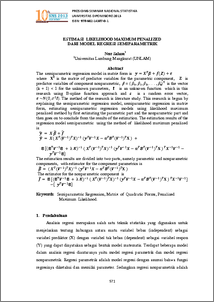# ESTIMASI LIKELIHOOD MAXIMUM PENALIZED DARI MODEL REGRESI SEMIPARAMETRIK

Salam, Nur (2013) ESTIMASI LIKELIHOOD MAXIMUM PENALIZED DARI MODEL REGRESI SEMIPARAMETRIK. PROSIDING SEMINAR NASIONAL STATISTIKA UNIVERSITAS DIPONEGORO 2013 . pp. 571-582. ISSN ISBN: 978-602-14387-0-1Preview
PDF
970Kb

## Abstract

Abstract The semiparametric regression model in matrix form is y=X^T β+f(Z)+ε where XT is the matrix of predictor variables for the parametric component, Z is predictor variables of component nonparametric, β = ( β0, β1, β2, . . . , βk)T is the vector (k + 1) × 1 for the unknown parameters, f is an unknown function which in this research using B-spline function approach and ε is a random error vector, ε ~N(0,σ^2 I). The method of the research is literature study. This research is begun by explaining the semiparametric regression model, semiparametric regression in matrix form, estimating semiparametric regression models using likelihood maximum penalized method by first estimating the parametric part and the nonparametric part and then goes on to conclude from the results of the estimation. The estimation results of the regression model semiparametric using the method of likelihood maximum penalized is ( y) ̂ = X β ̂+f ̂ y ̂ = 〖〖X( X〗^T 〖〖(V〗^(-1))〗^T X)〗^(-1) 〖(y〗^T V^(-1) X-α^T B^T 〖〖(V〗^(-1))〗^T X ) + B [(B^T V^(-1) B + λ K)^(-1) 〖〖〖( X〗^T 〖〖(V〗^(-1))〗^T X)〗^(-1) 〖(y〗^T V^(-1) X-α^T B^T 〖〖(V〗^(-1))〗^T X ) 〗^T X^(-1) V^(-1)-〖 y〗^T V^(-1) B] The estimation results are divided into two parts, namely parametric and nonparametric components, with estimator for the component parametrics is β ̂= 〖〖( X〗^T 〖〖(V〗^(-1))〗^T X)〗^(-1) 〖(y〗^T V^(-1) X-α^T B^T 〖〖(V〗^(-1))〗^T X ) The estimator for the nonparametric component is f ̂= B [(B^T V^(-1) B + λ K)^(-1) 〖 〖〖( X〗^T 〖〖(V〗^(-1))〗^T X)〗^(-1) 〖(y〗^T V^(-1) X-α^T B^T 〖〖(V〗^(-1))〗^T X ) 〗^T X^(-1) V^(-1) ] -[〖 y〗^T V^(-1) B] Keywords: Semiparametric Regression, Matrix of Quadratic Forms, Penalized Maximum Likelihood.

Item Type: Article Q Science > Q Science (General) Faculty of Science and Mathematics > Department of Statistics 40367 INVALID USER 23 Oct 2013 08:31 23 Oct 2013 08:31

Repository Staff Only: item control page